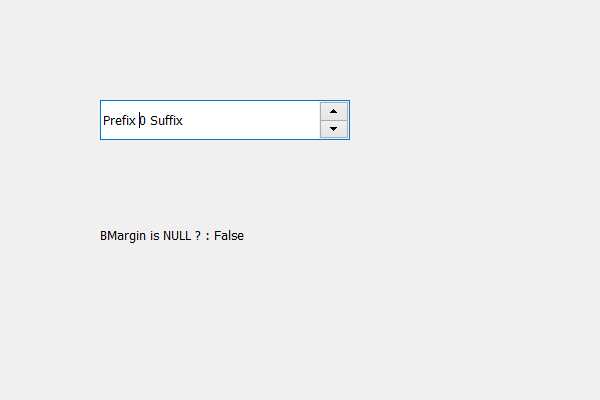Related Articles
PyQt5 QSpinBox – Checking if margin is NULL ?
• Last Updated : 19 May, 2020

In this article we will see how we can check if the margin of the spin box is NULL or not, by default it is set to zero although we can change this using `setContentsMargins` method with the spin box object. If any of the margin value is greater than 0 then spin box margin is not NULL.

Note : When each margin value is set to 0 then it means they are NULL

In order to do this we use isNull method.

Syntax : margin.isNull()
Here margin is the QMargin object of the spin box

Argument : It takes no argument

Return : It returns bool

Below is the implementation

 `# importing libraries``from` `PyQt5.QtWidgets ``import` `*` `from` `PyQt5 ``import` `QtCore, QtGui``from` `PyQt5.QtGui ``import` `*` `from` `PyQt5.QtCore ``import` `*` `import` `sys`` ` ` ` `class` `Window(QMainWindow):`` ` `    ``def` `__init__(``self``):``        ``super``().__init__()`` ` `        ``# setting title``        ``self``.setWindowTitle(``"Python "``)`` ` `        ``# setting geometry``        ``self``.setGeometry(``100``, ``100``, ``600``, ``400``)`` ` `        ``# calling method``        ``self``.UiComponents()`` ` `        ``# showing all the widgets``        ``self``.show()`` ` `        ``# method for widgets``    ``def` `UiComponents(``self``):``        ``# creating spin box``        ``self``.spin ``=` `QSpinBox(``self``)`` ` `        ``# setting geometry to spin box``        ``self``.spin.setGeometry(``100``, ``100``, ``250``, ``40``)`` ` `        ``# setting range to the spin box``        ``self``.spin.setRange(``0``, ``999999``)`` ` `        ``# setting prefix to spin``        ``self``.spin.setPrefix(``"Prefix "``)`` ` `        ``# setting suffix to spin``        ``self``.spin.setSuffix(``" Suffix"``)`` ` `        ``# creating label``        ``label ``=` `QLabel(``self``)`` ` `        ``# setting geometry to the label``        ``label.setGeometry(``100``, ``200``, ``200``, ``70``)`` ` `        ``# making it multi line label``        ``label.setWordWrap(``True``)`` ` `        ``# setting content margins``        ``self``.spin.setContentsMargins(``1``, ``0``, ``0``, ``0``)`` ` `        ``# getting content margins``        ``margin ``=` `self``.spin.contentsMargins()`` ` `        ``# checking if margin is NULL``        ``check ``=` `margin.isNull()`` ` `        ``# setting text to the label``        ``label.setText(``"BMargin is NULL ? : "` `+` `str``(check))`` ` `# create pyqt5 app``App ``=` `QApplication(sys.argv)`` ` `# create the instance of our Window``window ``=` `Window()``window.spin.setFocus()``# start the app``sys.exit(App.``exec``())`

Output :Attention geek! Strengthen your foundations with the Python Programming Foundation Course and learn the basics.

To begin with, your interview preparations Enhance your Data Structures concepts with the Python DS Course. And to begin with your Machine Learning Journey, join the Machine Learning – Basic Level Course

My Personal Notes arrow_drop_up